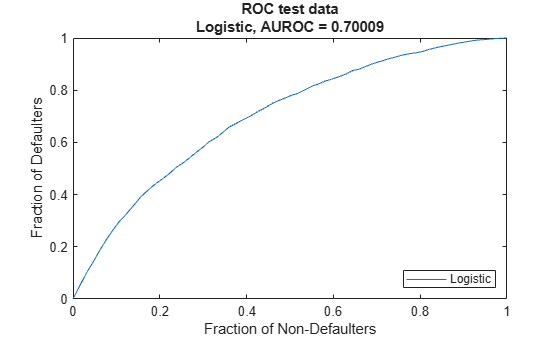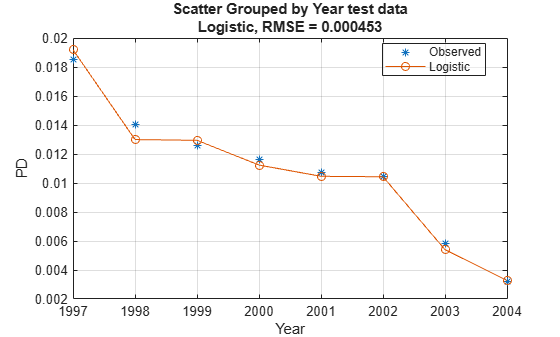# Logistic

Create `Logistic` model object for lifetime probability of default

## Description

Create and analyze a `Logistic` model object to calculate the lifetime probability (PD) of default using this workflow:

1. Use `fitLifetimePDModel` to create a `Logistic` model object.

2. Use `predict` to predict the conditional PD and `predictLifetime` to predict the lifetime PD.

3. Use `modelDiscrimination` to return AUROC and ROC data. You can plot the results using `modelDiscriminationPlot`.

4. Use `modelAccuracy` to return the RMSE of the observed and predicted PD data. You can plot the results using `modelAccuracyPlot`.

## Creation

### Syntax

``LogisticPDModel = fitLifetimePDModel(data,ModelType)``
``LogisticPDModel = fitLifetimePDModel(___,Name,Value)``

### Description

example

````LogisticPDModel = fitLifetimePDModel(data,ModelType)` creates a `Logistic` PD model object.If you do not specify variable information for `IDVar`, `AgeVar`, `LoanVars`, `MacroVars`, and `ResponseVar`, then: `IDVar` is set to the first column in the `data` input.`LoanVars` is set to include all columns from the second to the second-to-last columns of the `data` input.`ResponseVar` is set to the last column in the `data` input. ```

example

````LogisticPDModel = fitLifetimePDModel(___,Name,Value)` specifies options using one or more name-value pair arguments in addition to the input arguments in the previous syntax. The optional name-value pair arguments set model object properties. For example, ```LogisticPDModel = fitLifetimePDModel(data(TrainDataInd,:),"Logistic",'ModelID',"Logistic_A",'Description',"Logisitic_model",'AgeVar',"YOB",'IDVar',"ID",'LoanVars',"ScoreGroup",'MacroVars',{'GDP','Market'}'ResponseVar',"Default")``` creates a `LogisticPDModel` object using a `Logistic` model type. ```

### Input Arguments

expand all

Data, specified as a table, in panel data form. The `data` must contain an ID column. The response variable must be a binary variable with the value `0` or `1`, with `1` indicating default.

Data Types: `table`

Model type, specified as a string with the value of `"Logistic"` or a character vector with the value of `'Logistic'`.

Data Types: `char` | `string`

`Logistic` Name-Value Pair Arguments

Specify optional comma-separated pairs of `Name,Value` arguments. `Name` is the argument name and `Value` is the corresponding value. `Name` must appear inside quotes. You can specify several name and value pair arguments in any order as `Name1,Value1,...,NameN,ValueN`.

Example: ```LogisticPDModel = fitLifetimePDModel(data(TrainDataInd,:),"Logistic",'ModelID',"Logistic_A",'Description',"Logisitic_model",'AgeVar',"YOB",'IDVar',"ID",'LoanVars',"ScoreGroup",'MacroVars',{'GDP','Market'}'ResponseVar',"Default")```

User-defined model ID, specified as the comma-separated pair consisting of `'ModelID'` and a string or character vector. The software uses the `ModelID` to format outputs and is expected to be short.

Data Types: `string` | `char`

User-defined description for model, specified as the comma-separated pair consisting of `'Description'` and a string or character vector.

Data Types: `string` | `char`

ID variable indicating which column in `data` contains the loan or borrower ID, specified as the comma-separated pair consisting of `'IDVar'` and a string or character vector.

Data Types: `string` | `char`

Age variable indicating which column in `data` contains the loan age information, specified as the comma-separated pair consisting of `'AgeVar'` and a string or character vector.

Data Types: `string` | `char`

Loan variables indicating which column in `data` contains the loan-specific information, such as origination score or loan-to-value ratio, specified as the comma-separated pair consisting of `'LoanVars'` and a string array or cell array of character vectors.

Data Types: `string` | `cell`

Macro variables indicating which column in `data` contains the macroeconomic information, such as gross domestic product (GDP) growth or unemployment rate, specified as the comma-separated pair consisting of `'MacroVars'` and a string array or cell array of character vectors.

Data Types: `string` | `cell`

Variable indicating which column in `data` contains the response variable, specified as the comma-separated pair consisting of `'ResponseVar'` and a string or character vector.

Note

The response variable in the `data` must be a binary variable with `0` or `1` values, with `1` indicating default.

Data Types: `logical`

## Properties

expand all

User-defined model ID, returned as a string.

Data Types: `string`

User-defined description, returned as a string.

Data Types: `string`

Underlying statistical model, returned as a compact generalized linear model object. For more information, see `fitglm` and `CompactGeneralizedLinearModel`.

Data Types: `CompactGneralizedLinearModel`

ID variable indicating which column in `data` contains loan or borrower ID, returned as a string.

Data Types: `string`

Age variable indicating which column in `data` contains loan age information, returned as a string.

Data Types: `string`

Loan variables indicating which column in `data` contains loan-specific information, returned as a string array.

Data Types: `string`

Macro variables indicating which column in `data` contains macroeconomic information, returned as a string array.

Data Types: `string`

Variable indicating which column in `data` contains the response variable, returned as a string or character vector.

Data Types: `string`

## Object Functions

 `predict` Compute conditional PD `predictLifetime` Compute cumulative lifetime PD, marginal PD, and survival probability `modelDiscrimination` Compute AUROC and ROC data `modelAccuracy` Compute RMSE of predicted and observed PDs on grouped data `modelDiscriminationPlot` Plot ROC curve `modelAccuracyPlot` Plot observed default rates compared to predicted PDs on grouped data

## Examples

collapse all

This example shows how to use `fitLifetimePDModel` to create a `Logistic` model using credit and macroeconomic data.

```load RetailCreditPanelData.mat disp(head(data))```
``` ID ScoreGroup YOB Default Year __ __________ ___ _______ ____ 1 Low Risk 1 0 1997 1 Low Risk 2 0 1998 1 Low Risk 3 0 1999 1 Low Risk 4 0 2000 1 Low Risk 5 0 2001 1 Low Risk 6 0 2002 1 Low Risk 7 0 2003 1 Low Risk 8 0 2004 ```
`disp(head(dataMacro))`
``` Year GDP Market ____ _____ ______ 1997 2.72 7.61 1998 3.57 26.24 1999 2.86 18.1 2000 2.43 3.19 2001 1.26 -10.51 2002 -0.59 -22.95 2003 0.63 2.78 2004 1.85 9.48 ```

Join the two data components into a single data set.

```data = join(data,dataMacro); disp(head(data))```
``` ID ScoreGroup YOB Default Year GDP Market __ __________ ___ _______ ____ _____ ______ 1 Low Risk 1 0 1997 2.72 7.61 1 Low Risk 2 0 1998 3.57 26.24 1 Low Risk 3 0 1999 2.86 18.1 1 Low Risk 4 0 2000 2.43 3.19 1 Low Risk 5 0 2001 1.26 -10.51 1 Low Risk 6 0 2002 -0.59 -22.95 1 Low Risk 7 0 2003 0.63 2.78 1 Low Risk 8 0 2004 1.85 9.48 ```

Partition Data

Separate the data into training and test partitions.

```nIDs = max(data.ID); uniqueIDs = unique(data.ID); rng('default'); % for reproducibility c = cvpartition(nIDs,'HoldOut',0.4); TrainIDInd = training(c); TestIDInd = test(c); TrainDataInd = ismember(data.ID,uniqueIDs(TrainIDInd)); TestDataInd = ismember(data.ID,uniqueIDs(TestIDInd));```

Create `Logistic` Lifetime PD Model

Use `fitLifetimePDModel` to create a `Logistic` model using the training data.

```pdModel = fitLifetimePDModel(data(TrainDataInd,:),"Logistic",... 'AgeVar','YOB',... 'IDVar','ID',... 'LoanVars','ScoreGroup',... 'MacroVars',{'GDP','Market'},... 'ResponseVar','Default'); disp(pdModel)```
``` Logistic with properties: ModelID: "Logistic" Description: "" Model: [1x1 classreg.regr.CompactGeneralizedLinearModel] IDVar: "ID" AgeVar: "YOB" LoanVars: "ScoreGroup" MacroVars: ["GDP" "Market"] ResponseVar: "Default" ```

Display the underlying model.

`disp(pdModel.Model)`
```Compact generalized linear regression model: logit(Default) ~ 1 + ScoreGroup + YOB + GDP + Market Distribution = Binomial Estimated Coefficients: Estimate SE tStat pValue __________ _________ _______ ___________ (Intercept) -2.7422 0.10136 -27.054 3.408e-161 ScoreGroup_Medium Risk -0.68968 0.037286 -18.497 2.1894e-76 ScoreGroup_Low Risk -1.2587 0.045451 -27.693 8.4736e-169 YOB -0.30894 0.013587 -22.738 1.8738e-114 GDP -0.11111 0.039673 -2.8006 0.0051008 Market -0.0083659 0.0028358 -2.9502 0.0031761 388097 observations, 388091 error degrees of freedom Dispersion: 1 Chi^2-statistic vs. constant model: 1.85e+03, p-value = 0 ```

Use the `predict` function to predict conditional PD values. The prediction is a row-by-row prediction.

```dataCustomer1 = data(1:8,:); CondPD = predict(pdModel,dataCustomer1)```
```CondPD = 8×1 0.0092 0.0053 0.0045 0.0039 0.0037 0.0037 0.0019 0.0012 ```

Use `predictLifetime` to predict the lifetime cumulative PD values (computing marginal and survival PD values is also supported). The `predictLifetime` function uses the ID variable (see the `'IDVar'` property for the `Logistic` object) to transform conditional PDs to cumulative PDs for each ID.

`LifetimePD = predictLifetime(pdModel,dataCustomer1)`
```LifetimePD = 8×1 0.0092 0.0145 0.0189 0.0228 0.0264 0.0300 0.0319 0.0330 ```

Validate Model

Use `modelDiscrimination` to measure the ranking of customers by PD.

```DiscMeasure = modelDiscrimination(pdModel,data(TestDataInd,:),'DataID','test data'); disp(DiscMeasure)```
``` AUROC _______ Logistic, test data 0.70009 ```

Use `modelDiscriminationPlot` to visualize the ROC curve.

`modelDiscriminationPlot(pdModel,data(TestDataInd,:),'DataID','test data');`Use `modelAccuracy` to measure the accuracy of the predicted PD values, also known as model calibration. The `modelAccuracy` function requires a grouping variable and compares the accuracy of the observed default rate in the group with the average predicted PD for the group. For example, you can group by calendar year using the `'Year'` variable.

```AccMeasure = modelAccuracy(pdModel,data(TestDataInd,:),'Year','DataID','test data'); disp(AccMeasure)```
``` RMSE ________ Logistic, grouped by Year, test data 0.000453 ```

Use `modelAccuracyPlot` to visualize the observed default rates compared to the predicted probabilities of default (PD).

`modelAccuracyPlot(pdModel,data(TestDataInd,:),'Year','DataID','test data');`expand all

 Baesens, Bart, Daniel Roesch, and Harald Scheule. Credit Risk Analytics: Measurement Techniques, Applications, and Examples in SAS. Wiley, 2016.

 Bellini, Tiziano. IFRS 9 and CECL Credit Risk Modelling and Validation: A Practical Guide with Examples Worked in R and SAS. San Diego, CA: Elsevier, 2019.

 Breeden, Joseph. Living with CECL: The Modeling Dictionary. Santa Fe, NM: Prescient Models LLC, 2018.

 Roesch, Daniel and Harald Scheule. Deep Credit Risk: Machine Learning with Python. Independently published, 2020.